# Getting Started with ggplot2 in R

## Grammer

A grammar provides a foundation for understanding diffrent types of graphics. A grammar may also help us on what a well-formed or correct graphic looks like, but there will still be many grammatically correct but nonsensical graphics. This is easy to see by analogy to the English language: good grammar is just the first step in creating a good sentence.

## Grammar of Graphics

A grammar of graphics is a tool that enables us to clearly describe the components of a graphics. Such a grammar allows us to move beyond named graphics (e.g., the “scatterplot”) and gain insights into the deep structures that underlies the statistical graphics. ggplot2 proposes an alternative parameterization of the grammar, based around the idea of building up a graphic from multiple layers of data.

## Components of ggplot2

• Data and aesthetic mappings
• Geometric objects
• Scale
• Facet Specification
• Statistical Transformation
• Coordinate Syatem

## Layered grammar of graphics

Together, the data, mappings, statistical transformations and geometric objects form a layer. Plot may have different layers. Layers are responsible for creating the objects that we expect on the plots.

## How to use ggplot2 in R?

For this we need to have installed ggplot2 package in our IDE. Let us use ggplot in R builtin data diamonds.

library(ggplot2)
ggplot(diamonds, aes(carat,price)) + geom_point()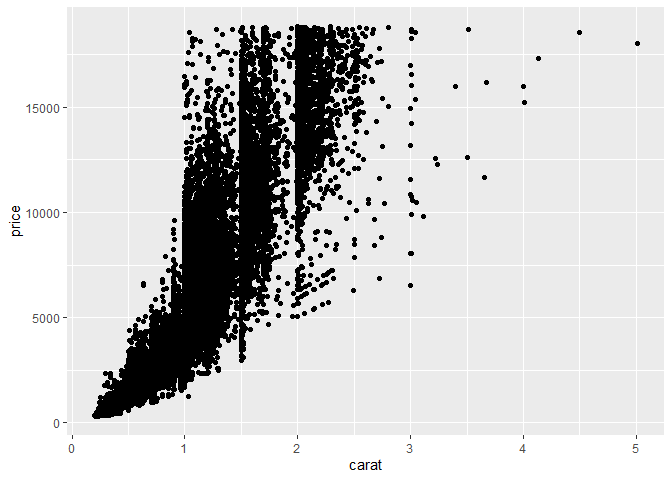geom_point is used for scatter plot. From above figure, we can see that whenever diamond’s carat increases, prices also increases. We can not see how the data distibuted for this, let’s make some changes in our code.

ggplot(diamonds,aes(carat,price)) + geom_point() +
scale_x_continuous() + scale_y_continuous()We can see better distribution of points than previous plot. We can clearly see that carat and price variable are not linearly distributed. To make it linearly distributable, lets make some changes in our code.

ggplot(diamonds, aes(carat,price)) + geom_point() +
stat_smooth(method = lm) + scale_x_log10() + scale_y_log10()

## geom_smooth() using formula 'y ~ x'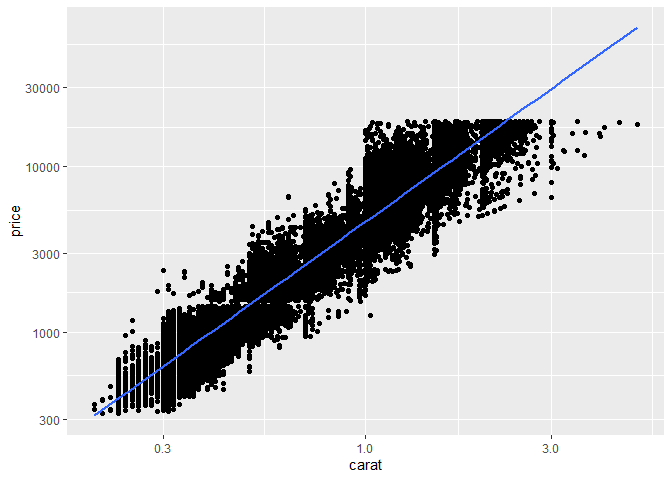From above graph, relationship between price and carat variables is linear. If we try the code without stat_smooth(method= lm) we can not see linear line in graph. Where lm means linear model.

ggplot(diamonds, aes(carat,price)) + geom_point() +
scale_x_log10() + scale_y_log10()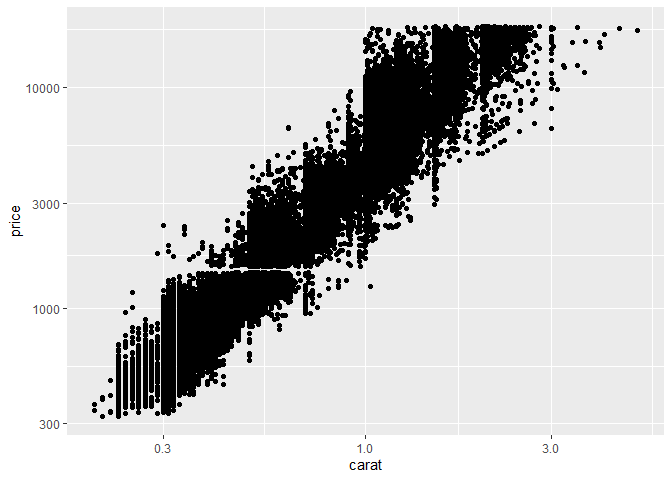## Lets make histogram of diamonds data

ggplot(diamonds, aes(price)) + geom_histogram()

## stat_bin() using bins = 30. Pick better value with binwidth.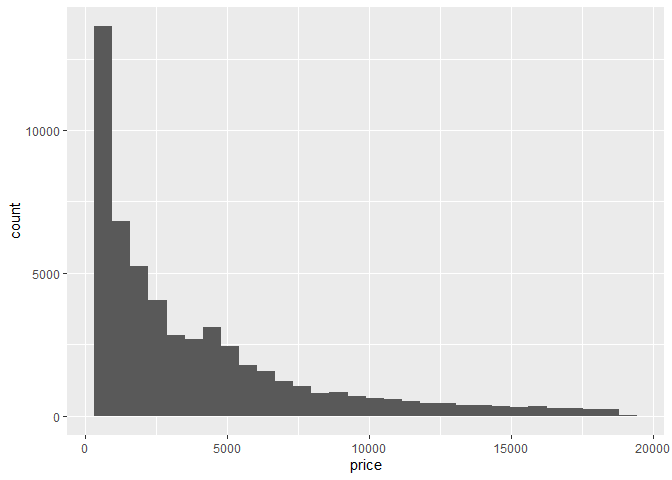To build histogram, we use function geom_histogram(). We should note that histogram is made on one dimensional data. If we want to add title of the plot we can do as,

ggplot(diamonds, aes(price)) + geom_histogram() + ggtitle("ggplot2 Histogram")

## stat_bin() using bins = 30. Pick better value with binwidth.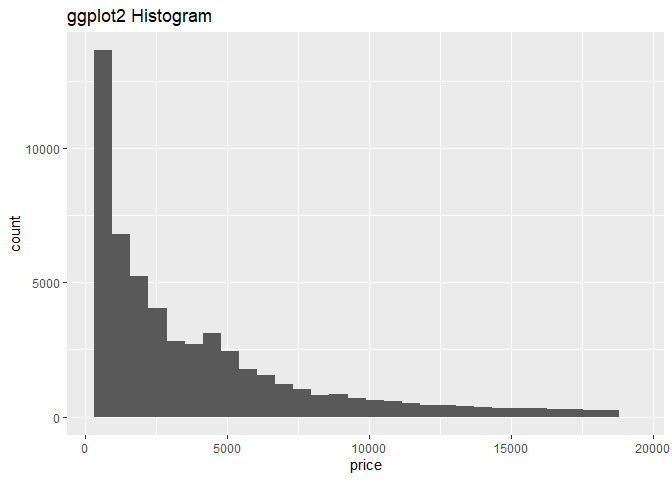## Let us try some other ggplot2 features in R builtin data mtcars

ggplot(mpg, aes(x = displ, y = hwy)) +
geom_point()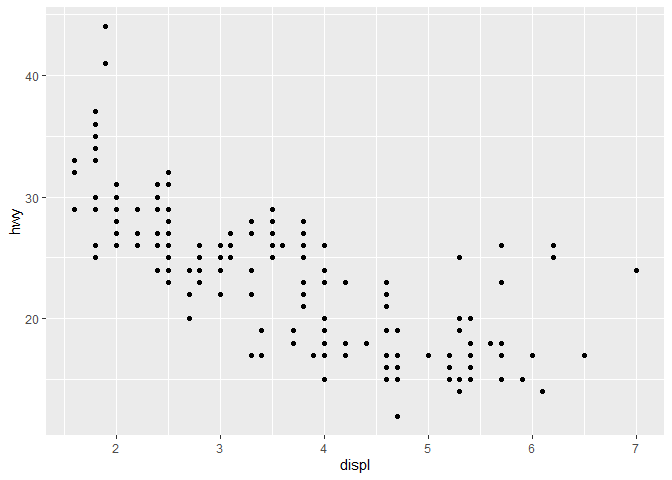The figure above shows scatterplot betweenhwyanddisplvariables of mtcars data from figure we can see as the values ofhwyincreases, values ofdisplvariable slightly decreases.

## Let’s add geam_smooth(): What will happen?

ggplot(mpg, aes(displ, hwy)) + geom_point() + geom_smooth()

## geom_smooth() using method = 'loess' and formula 'y ~ x'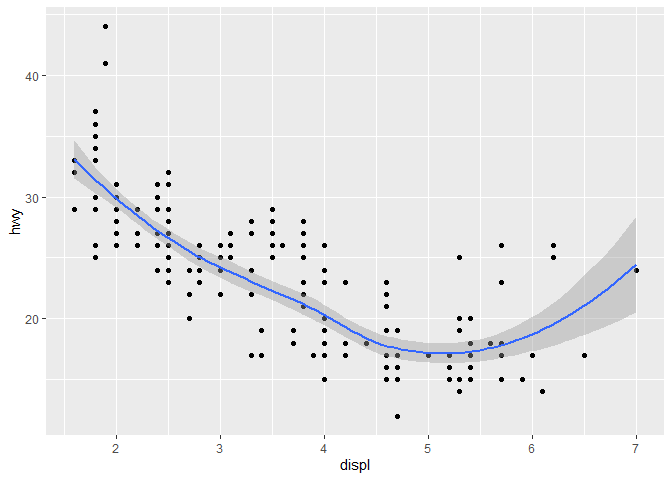We see there is smooth line appearing on the middle of the data points.

## Adding “wiggliness” in the smoothing plot

ggplot(mpg, aes(displ, hwy)) + geom_point() + geom_smooth(span = 0.2)

## geom_smooth() using method = 'loess' and formula 'y ~ x'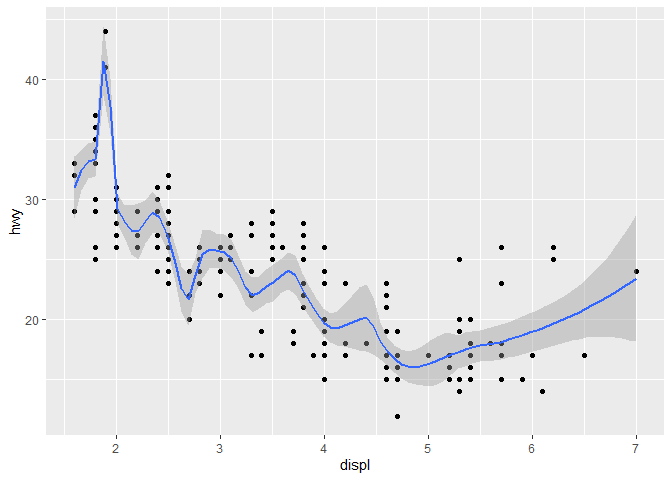What changes can we see in above graph and previous graph. Let us again check by keeping span = 1.

ggplot(mpg, aes(displ, hwy)) + geom_point()+ geom_smooth(span = 1)

## geom_smooth() using method = 'loess' and formula 'y ~ x'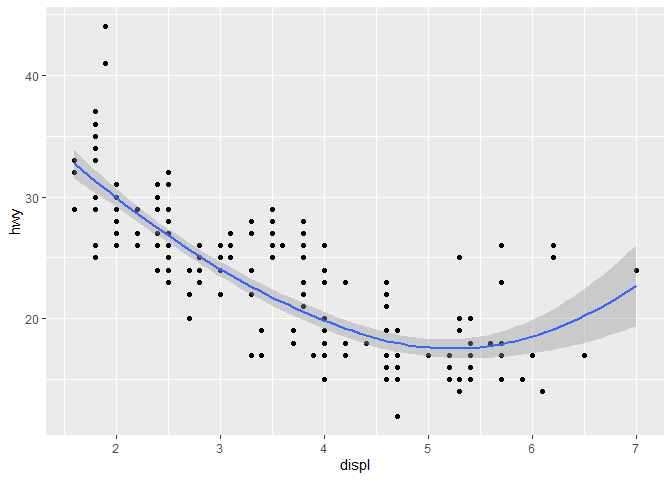We can make sense that, by default ggplot kept value of span 1. If we set method= lm inside geom_smooth() we can find stright smooth line. Let us try.

ggplot(mpg, aes(displ, hwy)) + geom_point() + geom_smooth(method = lm)

## geom_smooth() using formula 'y ~ x'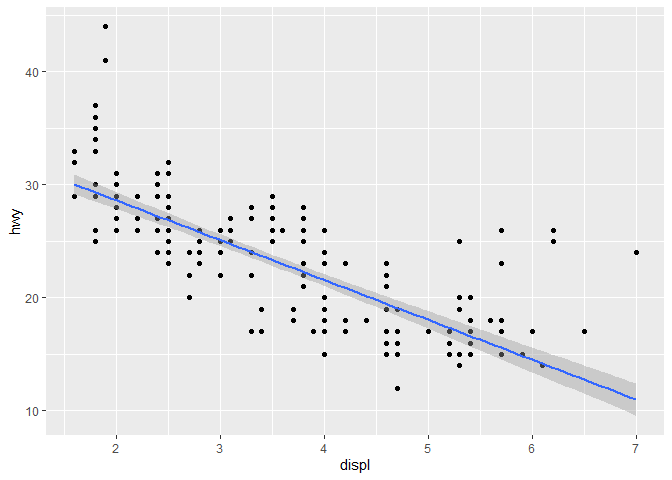## Let Modify our code little,

ggplot(mpg, aes(displ, hwy)) + geom_point() + geom_smooth(method = lm, se= FALSE)

## geom_smooth() using formula 'y ~ x'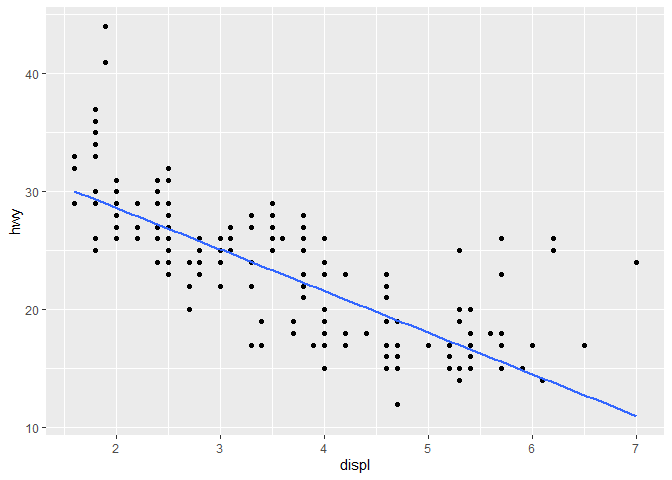By se= FALSE we added a smooth line.

## Fixed color

ggplot(mpg, aes(displ,hwy)) + geom_point(color = 'red')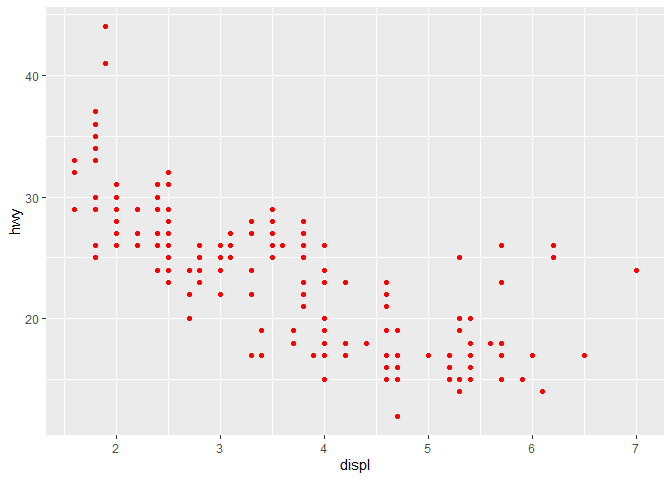## Changing color by variable attributes

Lets change our color based on class.

ggplot(mpg, aes(displ, hwy, colour = class)) +
geom_point()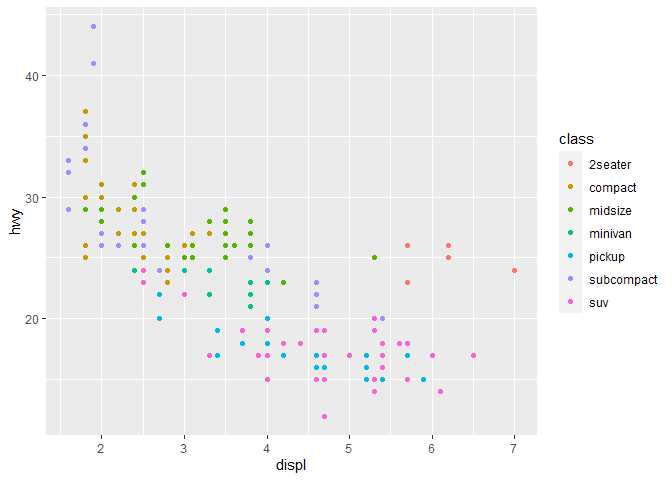Here we gave colors according to variable’s name.

## Getting multiple scatterplot of attributes

We can get multiple scatterplot by using facet_wrap() function.

ggplot(mpg, aes(displ, hwy)) + geom_point() +
facet_wrap(~class)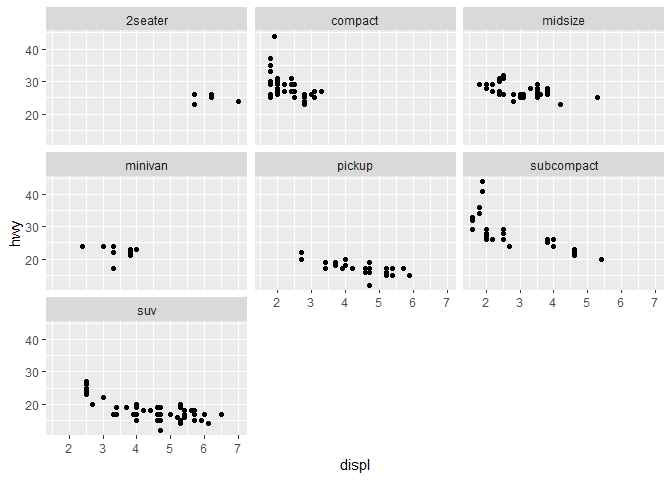In above figure, we found distribution of various variables along with displ and hwy variables.

## Histogram

ggplot(mpg, aes(hwy)) + geom_histogram()

## stat_bin() using bins = 30. Pick better value with binwidth.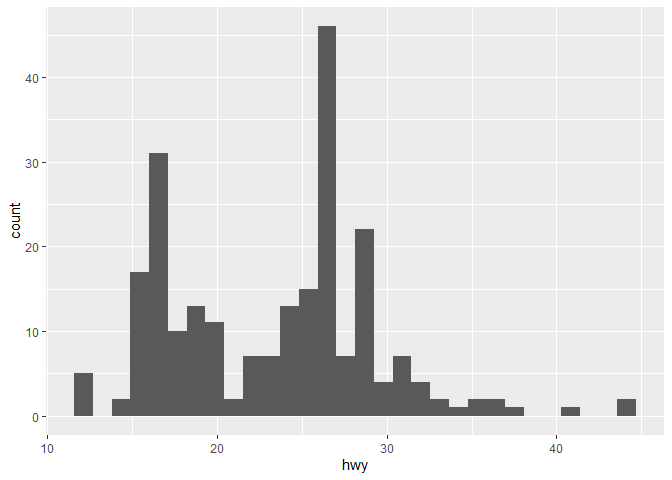hwy variable bins automatically.

## Changing bin size of the histogram

ggplot(mpg, aes(hwy)) + geom_histogram(binwidth = 2.5)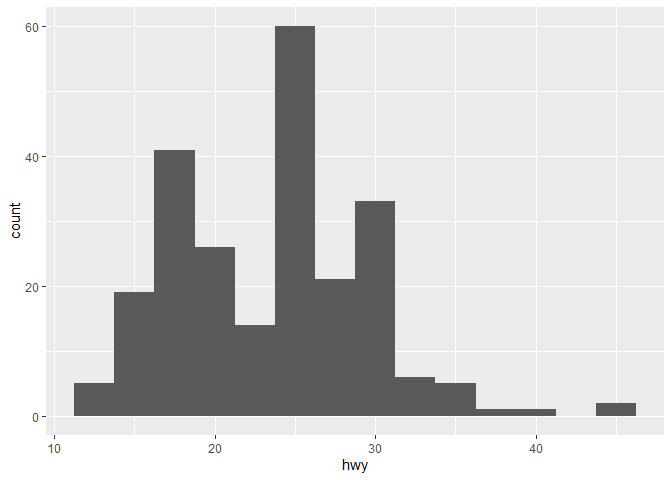## Frequency polygon

A frequency polygon is a line graph of class frequency plotted against class midpoint. It can be obtained by joining the midpoints of the top of the rectangles in the histogram.

ggplot(mpg, aes(hwy)) + geom_freqpoly()

## stat_bin() using bins = 30. Pick better value with binwidth.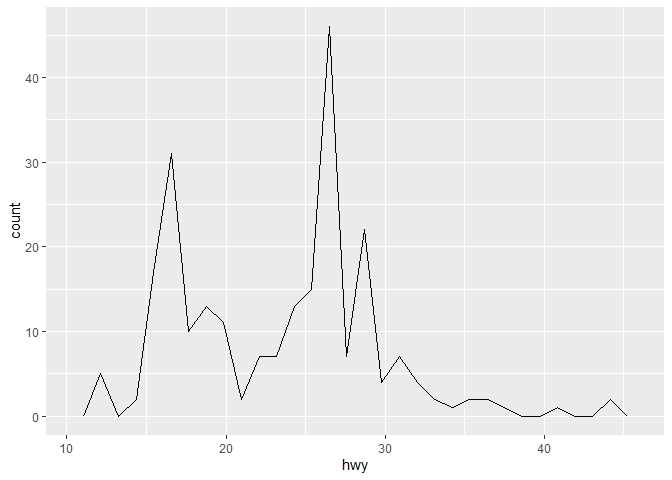## Change Bin size of frequency Polygon

ggplot(mpg, aes(hwy)) + geom_freqpoly(binwidth= 1)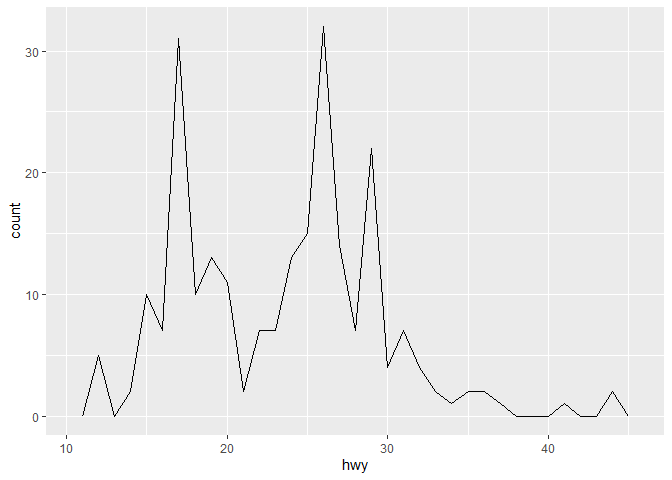We can see the effect of binwidth from figure by comparing above figure with previous one.

## Histogram with faceting:

We have already discussed about what a facet does in scatter plot. Similarly, in histogram it gives multiple subplots.

ggplot(mpg, aes(displ, fill = drv)) + geom_histogram(binwidth = 0.5) +
facet_wrap(~drv, ncol = 1)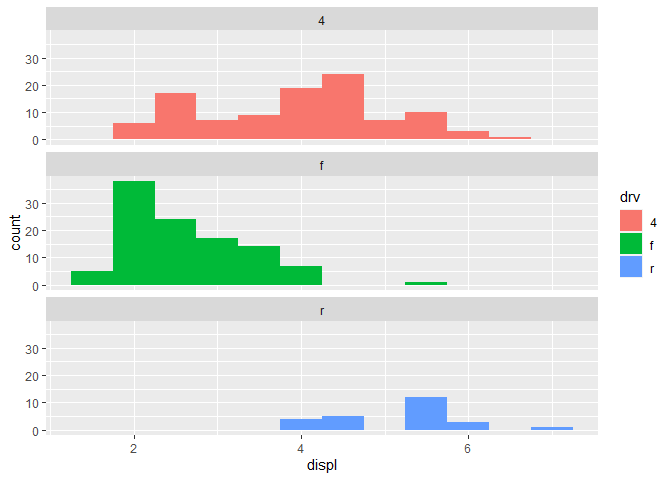## Bar plot

ggplot(mpg, aes(manufacturer)) + geom_bar()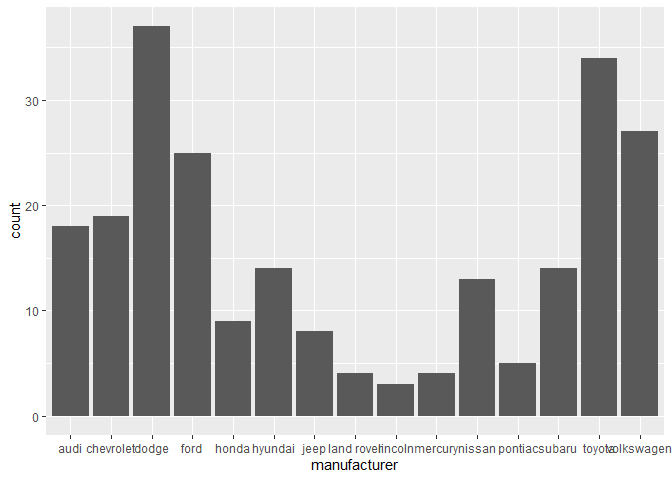We can draw bar plot in geom_bar() function. From bar plot we can see dodge and toyotao has maximum frequency.

## Let’s Use alpha inside geom_point()

ggplot(mpg, aes(cty, hwy)) + geom_point(alpha = 1 / 3)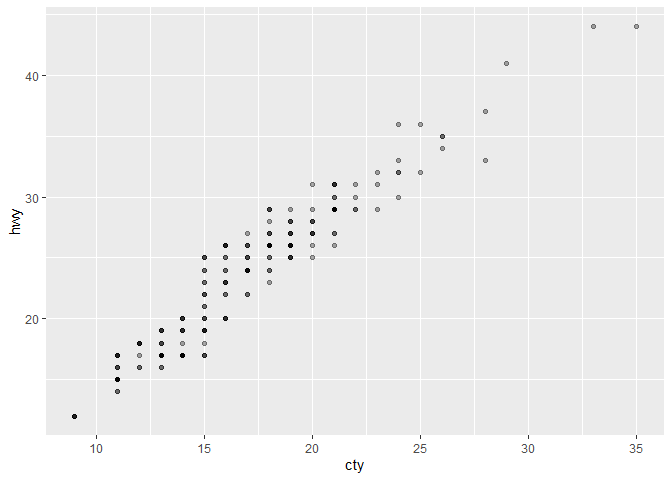Alpha refers to the opacity of a geom. Values of alpha range from 0 to 1, with lower values corresponding to more transparent colors.

## Modifying the axes

ggplot(mpg, aes(cty, hwy)) +geom_point(alpha = 1 / 3) + xlab("city driving (mpg)") +
ylab("highway driving (mpg)")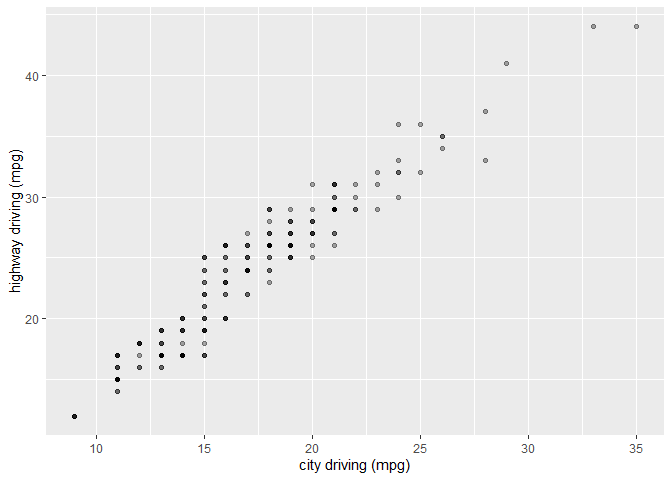ggplot(mpg, aes(cty, hwy)) + geom_point(alpha = 1 / 3) + xlab(NULL) +
ylab(NULL)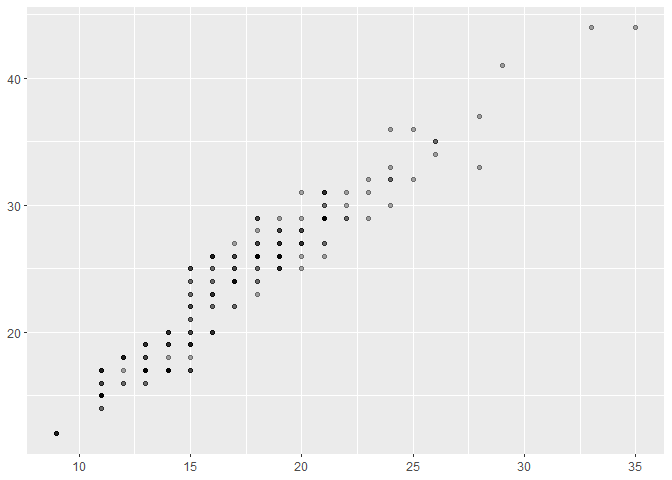Thats all for this part, thank you so much for reading.

Tags:

Categories:

Updated: